Technical Report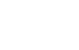Damping materials and their performance measurement
(page14)

3. The vibration velocity response and linearity for electroMagnetic Detector

The measurement diagram is shown in Figure 8.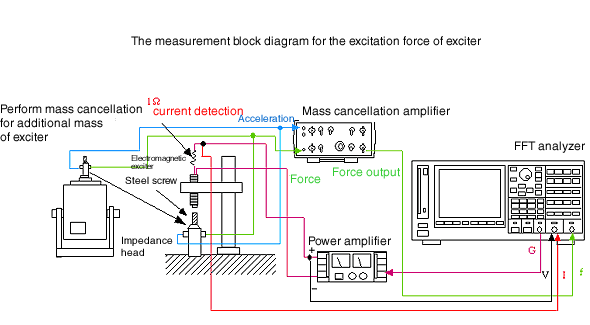Fig.8

The mass cancelation for additional mass is performed by the exciter beforehand, and the mechanical system is configured as shown in the figure. Use the same electroMagnetic Detector. Measure output of impedance head, current. The excitation force is different as MP-910, MP-912 has different gaps. Normalize the horizontal axis with the maximum voltage value. Normalize the maximum output value of the impedance head.

Frequency characteristic

The two types of exciter’s force response is shown in Figure 9, the current response is shown in Figure 10, and the current/force of frequency response function is shown in Figure 11.
MP-910 with 1 kΩ dC resistance is affected by its own impedance and its force response shows the bottom at about 8kHz, while the current/force of frequency response function shows the peak.
MP-912 with 100 Ω dC resistance shows relatively straightforward characteristics in the 10kHz range.
It finds that current vs voltage response of electromagnetic exciter shows more symmetrical with resonance frequency than input voltage vs response voltage of electromagnetic exciter. (Figure 12)
The excitation force cannot be read from the figure as it is normalized and, but the excitation force is about 0. 05 N / W (att1mmGAP, 1kHz). In order to obtain a practical force of 0.5N, MP-910 requires about 100V and MP-912 requires about 38V.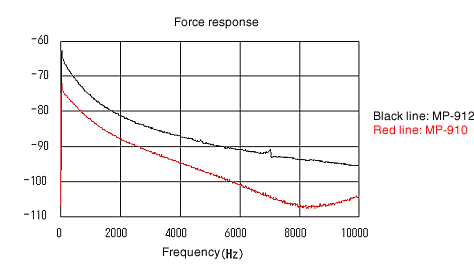Fig.9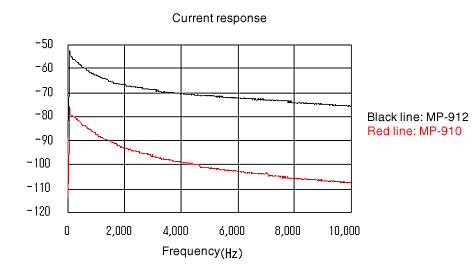Fig.10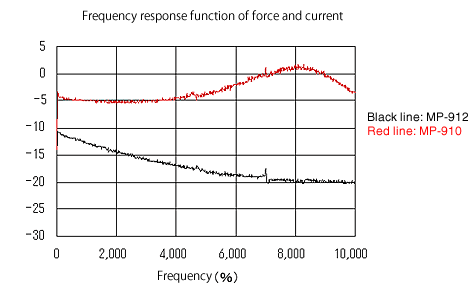Fig.11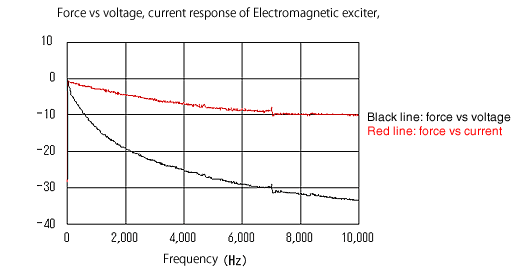Fig.12

Excitation linearity

Increase voltage gradually to the electromagnetic exciter and measure excitation linearity.
Its characteristics show in Figure 13 to 16. It finds them quite linearity.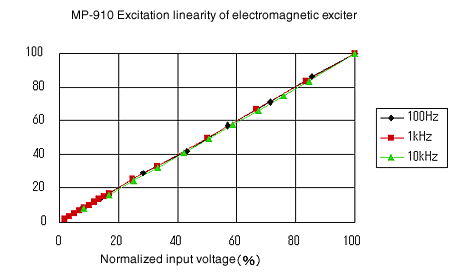Fig.13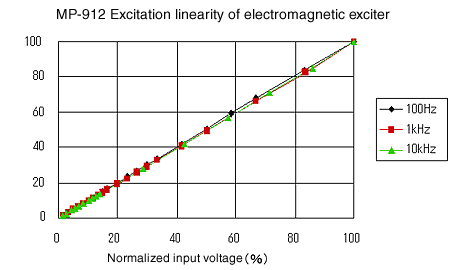Fig.14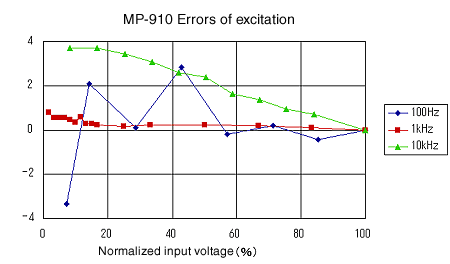Fig.15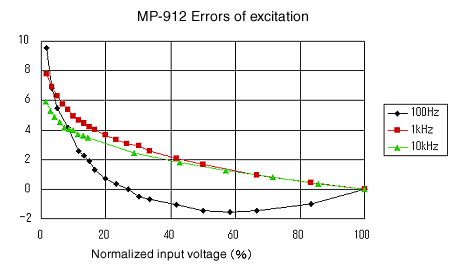Fig.16

4. Conclusion

Although the electroMagnetic Detector / exciter are frequently used in the cantilever method, but their characteristics have not been measured so far.

This measurement is performed from the question of whether the force is actually measured to input to the electromagnetic exciter as a quotient.

As a result, it is found that the "force" is well measured by measuring the input current to the electromagnetic exciter than measuring the voltage.

The accuracy of velocity detection, velocity occurrence are more high as expected. We measure our electroMagnetic Detector/ exciter this time, will measure other company’s ones.

As an electromagnetic exciter, more excitation force is likely to be obtained when many coils are wound, but this will increase the impedance and have an adverse effect.  It is the best thick wire should be wound appropriate times (about 1000 times) and the dC resistance should be several tens of Ω.